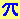World!Of
NumbersWON plate
37 |

Approximatingwith Palindromes

I found these four palindromes, we forget the decimal point for a while here,
in Eric Weisstein's Pi-pages (source Castellanos, D) :

1.09999901 x 1.19999911 x 1.39999931 x 1.69999961
=
3.141592 573...
which is a very good approximation of Pi - correct to six decimal places !
This approximation has recently been matched by Carlos Rivera
[ March 27, 1999 ] using the following 'palindrome' fraction :
 1633361 519915 = 3.141592 375... [Rank : 1,0833]

I soon understood that the lower the rank value the better the fraction approximates Pi.
For this ranking procedure now takes into account the total number of digits used.
Rank = (Average of numerator-digits and denominator-digits) / (Corresponding digits of Pi)
On [ April 25, 1999 ] I improved (the lower the better) Carlos' ranking
with the following fractions :
 666 212 = 3.1415 094... [Rank : 0,75]
The Number of the Beast 666, again, acquitted himself quite well !
The next fraction yields 13 corresponding digits with a rank of exactly 1 :
 6259909099526 1992590952991 = 3.1415926535897 878... [Rank : 1,00]

Pi fact from G. L. Honaker, Jr. :
In 1853 William Shanks published his calculations of pi to 707 decimal places.
Was he thinking Pi-lindromically ?
(Later it turned out that from place 528 onwards the computation was not correct!)

Approximating  e  with Palindromes

G. L. Honaker, Jr. recalls [ March 10, 1999 ]
Its best approximation with numbers less than 1.000 happens to be two palindromes ...
 878 323 = 2.7182 662... [Rank : 0,75]
which is a very good approximation of 'e' - correct to four decimal places !Bernard Leak (email) has some important comments [ September 8, 2004 ]

Approximating pi with palindromes: you forgot 22/7, which gets you 0.75 at once!
I'm surprised by your criterion. Surely being 'nearly right'
matters more than 'having so many decimal places correct' - thus,
36163/11511 = 3.141603... surely ought to do better than
96769/30803 = 3.141544... ... and it's more obvious to use the
magnitude of numerator for the other criterion ?
Ah, well, the die is cast. But I'd probably have wanted
to use something like (log(|pi - val|)/log(numerator))A000037Prime Curios!Prime PuzzleWikipedia 37Le Nombre 37Numberland 3737 Heaven```

```

[TOP OF PAGE]

Patrick De Geest - Belgium- Short Bio - Some Pictures